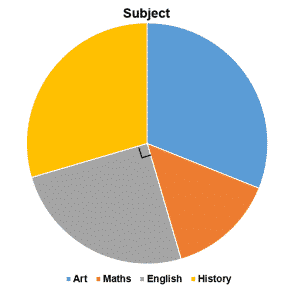# CSSE Essex 11+ Exam: Maths Practice Questions

The CSSE (or Consortium of Selective Schools in Essex) requires you to take two tests, one English and one Maths, for your 11+ exam.

Within this blog we will look at what the Maths test requires of you and additionally provide some example exam questions to enable a clearer understanding of the types of questions you may be asked on the day. The CSSE website also provides some sample exam papers.

You will be given sixty minutes to complete the maths paper. It is recommended that you answer all the questions you can in good time, and not spend too long on a question you are not sure on. This means that you leave yourself time at the end of the test to go over and try again on any questions you were unsure of the first time around. You will not be able to use a calculator during the test and you will be provided with space on the answer booklet to show your workings.

The test will include an array of different types of maths problems you will have studied at school. See below for our example questions to get an idea of the types of things you may be asked on the exam.

## CSSE Essex 11+ Exam: Maths Practice Example Questions:

1. Calculate the following: 962 + 1091

3. Multiply 250 by 12

4. Divide 210 by 5

For the following questions, there is one digit wrong in each calculation. They can be corrected by changing one digit in each question to the number 5. For the following questions, find out which digit should be replaced.

5. 87 – 62 = 29

6. 663 + 92 = 745

7. 84 + (41 x 3) = 219

Assuming that the letters of the alphabet have the following ascending values:

A = 1, B = 2, C = 3, D = 4 and so on, answer the following questions by adding the values of the words.

For example, the word DARK = 4 + 1 + 18 + 11 = a value of 34

8. What is the value of the word LIST?

9. Using the same letter value system, sort the following words in ascending order according to value (lowest to highest). Make sure to show in your workings out how you came to your answer.

• VOTE

• PINS

• WORD

10. How many grams are in 4.5kg?

11. Marsha has a piece of ribbon which measures 5m and 45cm long. She cuts off a long piece to wrap a present which measures 439cm. How long is the piece she now has remaining, in centimetres?

132 students were asked about their favourite subject. The following pie chart shows the results.12. What number of children chose English as their favourite subject?

13. Take a look at the following sweet shop price list:

White chocolate mice………….£0.20 each
Banana sweets…………………£0.13 each
Strawberry Laces………………£0.25 each
Liquorice Pieces……………….£0.16 each

Harry filled up a bag with sweets for him and his friend. In total he had 6 white mice, 7 banana sweets, 3 strawberry laces, and 5 liquorice pieces. He paid using a five pound note. How much change did he get back?

14. Six children took a literacy test. Their scores were as follows:

67, 60, 54, 49, 65, 55.

What was the mean (average) score to the closest whole number?

15. See below a table of values representing the formula 5 (y + 2). Some of the boxes have already been filled in. Using the values already in the table, fill in the missing gaps.## CSSE Essex 11+ Exam: Maths Practice Example Questions: Answers:

1. 2,053

2. 15.71

3. 3,000

4. 42

5. Last digit (9) should be 5

6. Second to last digit (4) should be 5

7. Fourth digit (1) should be 5

8. 12 + 9 + 19 + 20 = 60

9.Pins, word, vote
(Pins = 16 + 9 + 14 + 19 = 58)
(Word = 23 + 15 + 18 + 4 = 60)
(Vote = 22 + 15 + 20 + 5 = 62)

10. 4500 grams

11. 106 centimetres

12.33 children

13.(£1.20 + 0.91 + 0.75 + 0.80 = £3.66
£5.00 – 3.66 = 1.34)

14.58 (67 + 60 + 54 + 49 + 65 + 55 = 350 )

350 divided by 6 = 58.3 re-occurring so 58

15. Box 1: 70 (12 + 12 = 14, 14 x 5 = 70)

Box 2: 8 (8 + 2 = 10, 10 x 5 = 50)

*Please note that these questions are designed to give an idea of the types of questions that will be asked on the CSSE 11+ exam. These example questions do not correlate directly to the number or length of questions you will be required to answer on the real paper.

See our other blog on this topic here: CSSE Essex 11+ (Eleven Plus) – CSSE 2018 Entry – How2Become

Try your hand at some practice questions for the English part of the CSSE Essex 11 Plus on our other blog post: CSSE Essex 11+ Exam: English Practice Questions

Check out our YouTube Video on CSSE Essex 11 Plus Maths Practice Questions:

This site uses Akismet to reduce spam. Learn how your comment data is processed.